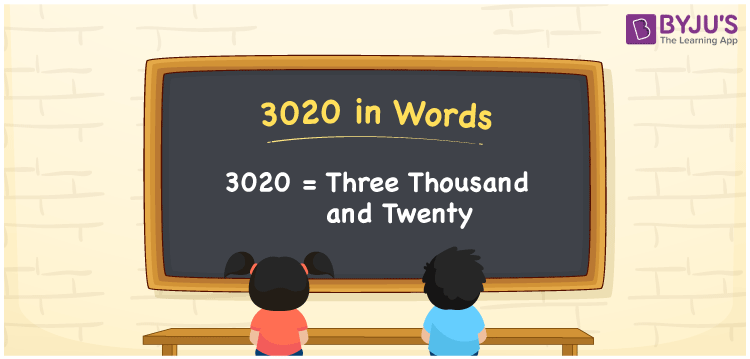# 3020 in Words

We can write 3020 in words as Three thousand and twenty. We can convert the number 3020 into words quickly with the help of a place value chart. The word form of 3020 is nothing but its number name. For instance, the number name for 3 is Three. Likewise, the number name for 3020 is Three thousand and twenty. In this article, you will understand how to derive the word form for 3020 in detail.

 3020 in words Three thousand and twenty Three thousand and twenty in Numbers 3020

## 3020 in English Words

We generally write numbers in words using the English alphabet. So, we spell 3020 in English as “Three thousand and twenty”.## How to Write 3020 in Words?

The below table displays the place values for all the four digits of the number 3020. So, let’s learn the conversion of 3020 to word form.

 Thousands Hundreds Tens Ones 3 0 2 0

Here, ones = 0, tens = 2, hundreds = 0, thousands = 3

These values can be written as:

3 × Thousand + 0 × Hundred + 2 × Ten + 0 × One

= 3 × 1000 + 0 × 100 + 2 × 10 + 0 × 1

= 3000 + 20

= Three thousand + Twenty

= Three thousand and twenty

Therefore, 3020 in words = Three thousand and twenty.

As we know, 3020 is a natural number that precedes 3021 and succeeds 3019.

3020 in words – Three thousand and twenty

Is 3020 an odd number? – No

Is 3020 an even number? – Yes

Is 3020 a perfect square number? – No

Is 3020 a perfect cube number? – No

Is 3020 a prime number? – No

Is 3020 a composite number? – Yes

## Frequently Asked Questions on 3020 in Words

Q1

### How do you write 3020 in words?

We can write the number 3020 in English words as Three thousand and twenty.
Q2

### How to write Rs. 3020 in words on a cheque?

On a cheque, we generally write an amount of Rs. 3020 in words as “Three thousand and twenty rupees only”.
Q3

### Write the answer for 3020 – 990 in words.

3020 – 990 = 2030 Therefore, the value of 3020 – 990, i.e., 2030 is written in words as Two thousand and thirty”.